1 Introduction

Recent developments in high-throughput technologies for studying biological systems enables the researcher to simultaneously obtain several different types of data (“omics”) over the course of an experiment. There exist many techniques for analysing the behaviour of these omics individually, but combining multiple classes of omics data can be used to give a better understanding of the biological system in question; the whole is greater than the sum of its parts. Integration of different types of omics data is an increasingly important technique for studying biological systems.

The first step in this kind of integration analysis is to identify patterns in data shared by all the omics classes, and use these patterns to identify outliers. The most common techniques for this sort of analysis are clustering and principal components analysis.

The STATegRa package provides several different techniques for the evaluation of reproducibility among samples and across experimental conditions by combining the information contained in multiple omics datasets. This is intended as a starting point for further integration analysis of any multi-omics dataset.

The STATegRa package implements two main utilities for this purpose: component analysis and clustering.

Component Analysis
Three different techniques for analysing the common and distinctive variability between two different, multi-omics datasets are provided, along with various utility functions and plotting tools to evaluate the results.
Clustering
Methods are provided to cluster together features across different omics types, with a view to finding interesting similarities between features (rather than similarities between samples).

Furthermore, the next important step is the identification of genes, which are differential expressed between the experimental conditions under study, according to the different data types considered as a whole. The STATegRa package provides the possibility to identify genes which are differential expressed in one or more data types. One main utility is implemented for this purpose: omicsNPC.

omicsNPC
omicsNPC is specifically devised for identifying differentially expressed genes by ‘holistically’ combining different omics data. It applies the NonParametric Combination (NPC) methodology (Pesarin and Salmaso 2010) in order to combine all available information by taking into account possible between-datasets correlations.

This guide provides an overview of the different techniques included in the package, some worked examples of using the tools and some guidance on interpretation of the results obtained.

2 Getting Started

The STATegRa package can be obtained from the Bioconductor repository.

Load the STATegRa package into an R session by typing:

General information about usage of the package and the algorithms used can be found in the package vignette. In addition, every public function in the package is documented and help can be found in the normal R fashion:

help(package="STATegRa") ## Package help
?omicsCompAnalysis ## Specific function help

3 Omics Component Analysis

3.1 Overview

The joint analysis of multiple omic datasets, both containing different classes of data and from different experimental conditions, could provide a “global” view on the biological system of interest. The major challenge in this type of analysis is to distinguish between the underlying mechanisms affecting all datasets, and the particular mechanisms which affect each omic dataset separately. Three different methods are provided to this end: DISCO-SCA (Van Deun et al. 2012; Schouteden et al. 2013, 2014), JIVE (Lock et al. 2013) and O2PLS (Trygg and Wold 2003). Each method provides the user with a decomposition of the variability of the composite data into common and distinctive variability. All of them are based on singular value decomposition (SVD) of the data matrix, however they use different models to accomplish this.

The DISCO-SCA (Van Deun et al. 2012) approach consists of two steps. First a Simultaneous Components Analysis (SCA) is performed, then the scores obtained are rotated into a DIStinctive and COmmon structure (hence, DISCO). Therefore, by applying SCA approach, each block of data $$X_k$$ of size $$I\times J_k$$ becomes

$X_k=TP_k^T+E_k$

with $$T$$ the $$I\times R$$ matrix of components scores that is shared between all blocks and $$P_k$$ the $$J_k\times R$$ matrix of components loadings for block $$k$$. Then, a rotation criterion is used where the target is the rotation which specifies distinctive components as components having zero scores in the positions that correspond to the data blocks the component does not underlie, and the remaining entries are arbitrary. The rotation matrix $$B$$ is found by minimizing $$min(B)||W \circ (P_{target}-[P_1^TP_2^T]B)||^2 \mbox{ such that } B^TB=I=BB^T$$, where $$W$$ is a binary matrix having ones in the positions of the entries in the target and zero elsewhere.

The JIVE approach (Lock et al. 2013) model is as following: Let $$X_1,X_2$$ be two blocks of data and $$X=[X_1,X_2]$$ represent the joint data, then the JIVE decomposition is defined as:

$X_i=J_i+A_i+\epsilon_i \mbox{, }i=1,2$

where $$J=[J_1,J_2]$$ is the $$p\times n$$ matrix of rank $$r<rank(X)$$ representing the joint structure, $$A_i$$ is the $$p_i\times n$$ matrix of rank $$r_i<rank(X_i)$$ representing the individual structure of $$X_i$$ and $$\epsilon_i$$ are $$p_i\times n$$ error matrices of independent entries.

Finally, the O2PLS (Trygg and Wold 2003) approach uses multiple linear regression to estimate the pure constituent profiles and divides the systematic part into two, one common to both blocks and one not. The O2PLS model can be written as a factor analysis where some factors are common between both blocks.

$\mbox{X model: } X=TW^T+T_{Y-ortho}P^T_{Y-ortho}+E \\ \mbox{Y model: } Y=UC^T+U_{X-ortho}P^T_{X-ortho}+F \\ \mbox{Inner relation: } U=T+H$

In this section, the different techniques for the analysis of the variability among different samples and conditions are explained. In addition, a worked example with an explanation of the interpretation of the graphical outputs is provided.

3.2 Usage

The typical workflow for component analysis is shown below.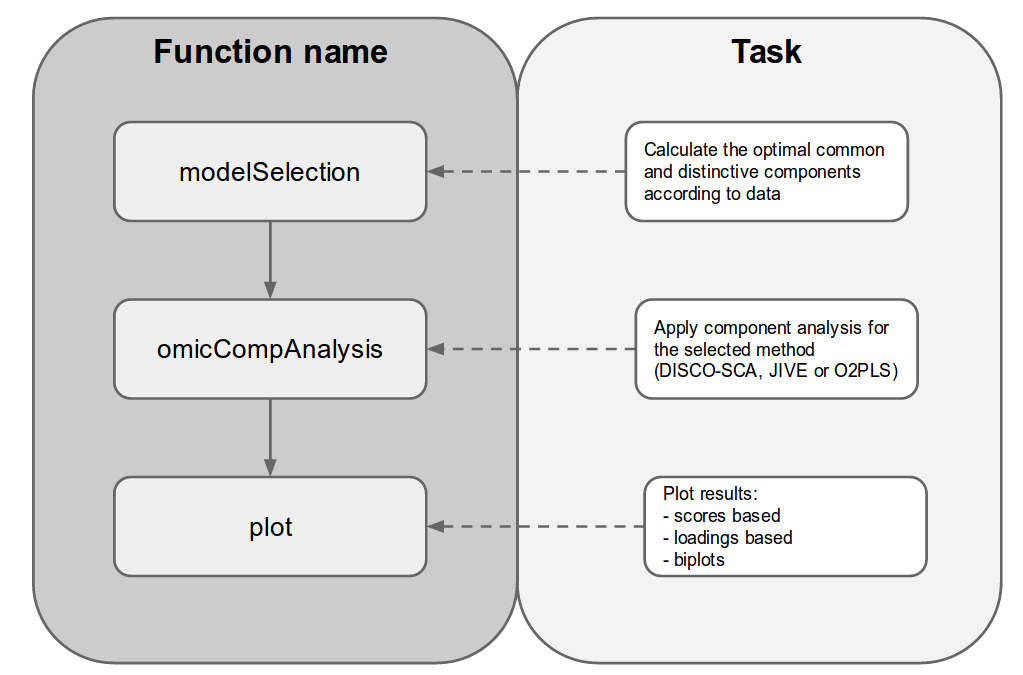Main steps in components analysis

Firstly, the number of common and distinctive components must be determined. The modelSelection() function (see Model Selection) provides a heuristic for this if the numbers are not known.

The main analysis is done by running the omicsCompAnalysis() function (see Component Analysis). The input data is a list of ExpressionSet objects, one for each block of data. These ExpressionSet objects can be created from a typical data matrix by using the createOmicsExpressionSet() function. An object describing the experiment design can also be added, which is used to appropriately format plots of the results. The omicsCompAnalysis() function allows the user to preprocess the input data by scaling and centering each block and/or weighting blocks together (to avoid the effects of blocks having different sizes). After the selected preprocessing is done, the analysis with the selected method is applied. The results are provided in a caClass object. This class contains the common and distinctive scores/loadings, as well as the initial data and the selected configuration for the analysis.

Finally, results can be plotted by using several plot functions on a caClass object (see Plot results).

3.3 Worked example

The dataset used for this section is based on the one used in OmicsClustering (STATegRa_S1), but is modified in a second step to obtain a dataset with a fixed number of common components by using the process described in (Van Deun et al. 2012). The initial dataset comprises $$23,293$$ genes and 534 miRNAs, but for the example presented here, 600 genes and 300 miRNAs are selecting according their significance in an ANOVA analysis comparing tumor subtypes1 Analysis done using the limma (Smyth 2005) R-package from Bioconductor.

Data can be loaded by typing:

data("STATegRa_S3")
ls()
##  "Block1.PCA" "Block2.PCA" "ed.PCA"     "g_legend"

The loaded data consists of two matrices (Block1.PCA, Block2.PCA) corresponding to gene and microRNA expression data respectively. Also, another matrix (ed.PCA) indicating the experimental design of the data is provided. This experimental design matrix is a one-column matrix indicating which subtype of tumour corresponds to each sample. For the main analysis, the input consists of a list of expression sets. These matrices could be easily converted in ExpressionSet objects by using createOmicsExpressionSet() function as follows:

# Block1 - gene expression data
B1 <- createOmicsExpressionSet(Data=Block1.PCA, pData=ed.PCA,

# Block2 - miRNA expression data
B2 <- createOmicsExpressionSet(Data=Block2.PCA, pData=ed.PCA,

3.3.2 Model Selection

To perform component analysis, it is required to find the number of common and distinctive components that the dataset is expected to contain. This step is optional; if you know how many common and distinctive components are expected, this can be used as input to the next step.

Model selection is done by using the modelSelection() function. This function first calculates the optimal number of common components. Then, the optimal number of individual components is found by subtracting the optimal number of individual components and the optimal number of common components calculated before. This individual components selection could be done by a cross-validation of the individual PCA results2 This option is not avaliable yet, but can be done using other R packages such as pcaMethods or missMDA, or by some other criteria.

For common components estimation, the Simultaneous Component Analysis (SCA) is applied. The idea is that the scores for both blocks should have a similar behavior if the components are in a common mode. That is, the estimation of the original data by using $$\hat{X}=T_XP'_X$$ and $$\hat{X}_Y=T_YP'_X$$ should give similar results. If not, the scores were calculated using uncommon factors and are not common components. To evaluate if a component is common or not, the ratios between the explained variances (SSQ) of each block and its estimation ($$SSQ_X/SSQ_{X_Y}$$ and $$SSQ_Y/SSQ_{Y_X}$$) are used. The highest component having its ratios between $$0.8$$ and $$1.5$$ is selected as the optimal number of common components. The SSQ can be shown as plots.

For distinctive components, function allows the user to select the optimal number of distinctive components depending on the percentage of accumulated variance explained, the individual explained variance of each component, the absolute value of its variability or just a fixed number of components.

Preprocessing of data can be done using this function, and should be specified by using the parameters center, scale and weight. If these parameters are not provided, preprocessing is not applied by default. Centering and scaling is applied independently to each block of data, while weight is applied to both blocks together. Weighting between blocks should be applied when the size of datasets is different.

ms <- modelSelection(Input=list(B1, B2), Rmax=3, fac.sel="single%",
varthreshold=0.03, center=TRUE, scale=TRUE,
weight=TRUE, plot_common=FALSE, plot_dist=FALSE)
## Common components
##  2
##
## Distinctive components
## []
##  2
##
## []
##  2
grid.arrange(ms$common$pssq, ms$common$pratios, ncol=2) #switching plot_common=TRUE gives automaticaly these plots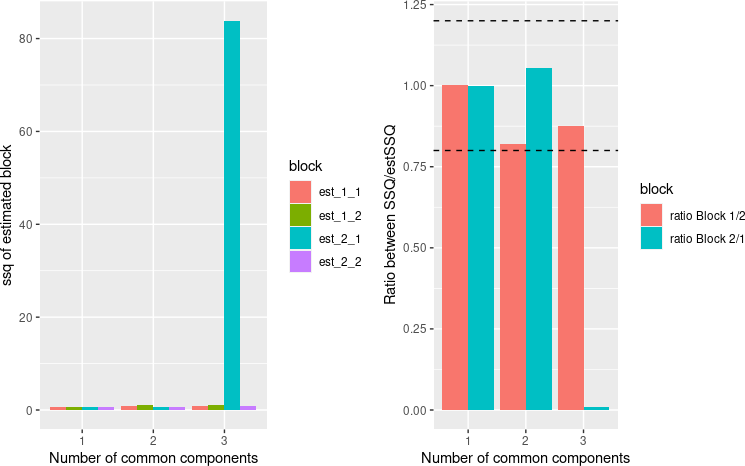This function automatically calculates the optimal number of common (allowing the visualization of SSQs) and individual components depending on the maximal number of common components and the individual components selection criteria provided by the user. The result is a list with the common and distinctive component analysis.

3.3.3 Component Analysis

Component Analysis is done using omicsCompAnalysis() function, where the method (DISCO, JIVE or O2PLS) to be applied is indicated via the method parameter. Preprocessing of data can be done using this function, and should be specified by using the parameters center, scale and weight, in the same way as modelSelection() function. If these parameters are not provided, preprocessing is not applied by default. Centering and scaling is applied independently to each block of data, while weight is applied to both blocks together. Weighting between blocks should be applied when the size of datasets is different. To use this function you have to specify the number of common and distinctive components (see Model Selection). Finally, the convThres and maxIter parameters are stop criteria for the DISCO-SCA and JIVE approaches.

discoRes <- omicsCompAnalysis(Input=list(B1, B2), Names=c("expr", "mirna"),
method="DISCOSCA", Rcommon=2, Rspecific=c(2, 2),
center=TRUE, scale=TRUE, weight=TRUE)
jiveRes <- omicsCompAnalysis(Input=list(B1, B2), Names=c("expr", "mirna"),
method="JIVE", Rcommon=2, Rspecific=c(2, 2),
center=TRUE, scale=TRUE, weight=TRUE)
o2plsRes <- omicsCompAnalysis(Input=list(B1, B2),Names=c("expr", "mirna"),
method="O2PLS", Rcommon=2, Rspecific=c(2, 2),
center=TRUE, scale=TRUE, weight=TRUE)

The results obtained are in a caClass object.

slotNames(discoRes)
##   "InitialData"   "Names"         "preprocessing" "preproData"
##   "caMethod"      "commonComps"   "distComps"     "scores"

Most of the slots in this class provide information about the input parameters/data of the function. The InitialData slot stores the input list of ExpressionSet objects and Names the specified names of the omics data sets. The preprocessing slot is a vector indicating the preprocessing applied to the data and preproData contains the so-processed data. The caMethod slot is a character indicating which components analysis method was applied to the data. The slots named commonComps and distComps indicate the number of common and distinctive components provided. Finally, the slots associated with the results of the analysis are scores, loadings and VAF. Finally, the others slot stores extra information specific to each different method.

These slots are accessible via accessor functions. All these functions allow the user to choose which part of the information in the slot is to be retrieved (e.g. the block of data whose information is retrieved can be specified in almost all accession functions). To access the initial data the getInitialData() and getMethodInfo() functions should be used. The first of these retrieves the initial data used for the component analysis and second one retrieves the method employed for the analysis as well as the number of common and distinctive components. The getPreprocessing() function allows the access to preprocessing information and preprocessed data. The results of the analysis can be displayed by using getScores(), getLoadings() and getVAF() functions. These functions allow the user to choose between common or individual results, as well as the block of data whose results are to be displayed.

The structure of scores and loadings data from DISCO-SCA and JIVE analyses are the same. Scores associated to common components are represented in a matrix with samples in the rows and as many columns as common components are selected. For O2PLS, these scores are instead divided into two matrices with the same structure, one associated to the contribution of the common components to one block and one associated to the other block. Scores associated to distinctive components are also represented by two matrices, one associated to each block, where rows represent samples and the number of columns depends of the number of distinctive components associated to each block. The loadings structure is the same for all methods. Two loadings matrices for the common part and two for the distinctive part, one associated to each block, are also provided.

# Exploring DISCO-SCA (or JIVE) score structure
getScores(discoRes, part="common")
getScores(discoRes, part="distinctive", block="1")
getScores(discoRes, part="distinctive", block="2")

# Exploring O2PLS score structure
getScores(o2plsRes, part="common", block="expr")
getScores(o2plsRes, part="common", block="mirna")
getScores(o2plsRes, part="distinctive", block="1")
getScores(o2plsRes, part="distinctive", block="2")

The variance explained for (VAF) each component is given in the VAF slot, as a list containing the VAF for common and distinctive components. In the case of O2PLS, VAF cannot be calculated, because the components are not orthogonal. VAF can be plotted by using the plotVAF() function. The structure of plots produced for a DISCO-SCA and JIVE result are different. In the case of DISCO-SCA, components of individual blocks have an associated error due to errors in the rotation. This is because the DISCO-SCA distinctive components have VAF in the other block. This VAF not associated to the corresponding block could be interpreted as the error for not having a perfect rotation3 See (Van Deun et al. 2012) for more details..

Example code and output for both approaches is shown below.

# DISCO-SCA plotVAF
getVAF(discoRes)
## $common ## Comp.1 Comp.2 ## Block1 0.5474047 0.17434917 ## Block2 0.6189476 0.09274787 ## ##$dist
## $dist$Block1
##     Comp.1     Comp.2
## 0.19869661 0.07953545
##
## $dist$Block2
##    Comp.1    Comp.2
## 0.1632240 0.1249982
##
## $dist$cross
##              Comp.1       Comp.2
## Block1 9.018248e-08 2.678459e-07
## Block2 1.271226e-07 4.562942e-07
plotVAF(discoRes)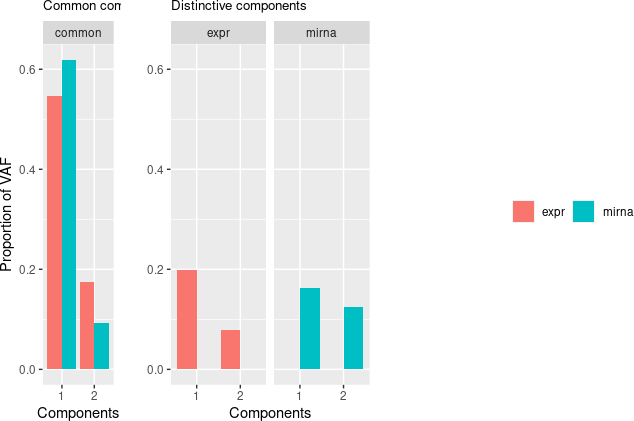# JIVE plotVAF
getVAF(jiveRes)
## $common ## common ## 1 0.8136602 ## 2 0.1863398 ## ##$dist
## $dist$Block1
##   distintive
## 1  0.7141268
## 2  0.2858732
##
## $dist$Block2
##   distintive
## 1  0.5662978
## 2  0.4337022
plotVAF(jiveRes)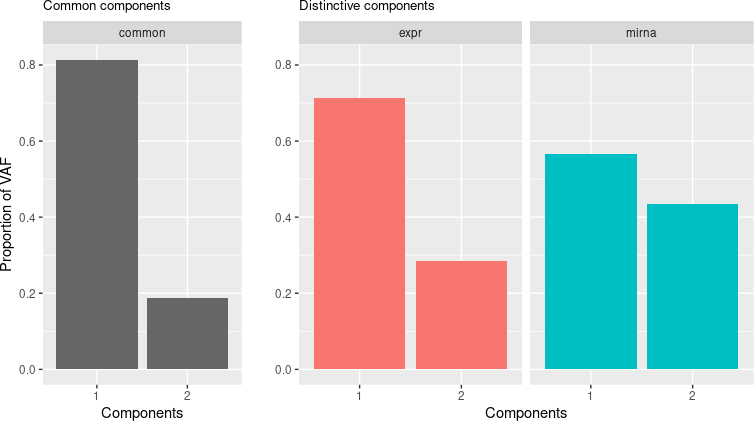3.3.4 Plot results

Plotting the results obtained from omicsCompAnalysis() can be done by using plotRes() function. plotRes() allows plotting of scores, loadings or both, for common and distinctive parts, as well as combined plots of both parts together.

The most important parameters for plotRes() are:

object
caClass object, usually a result from omicsCompAnalysis() function.

comps, components to plot. If combined=FALSE, it indicates the x and y components of the type and block chosen. If combined=TRUE, it indicates the component to plot for the first block of information and the component for the second block of information to plot together. By default the components are set to c(1,2) if combined=FALSE and to c(1,1) if combined=TRUE.

type=c("common", "individual", "both"). Are common or individual components had to be represented? If both a common and individual componanent are represented.

combined. Logical indicating if the plot is a simple plot representing two components from the same block of information, or a combined representation. The effect of the variable depends also on the values given for comps, block and type.

block indicates which block has to be represented. It can be specified by a numeric value (1 or 2) or a character (name of the block in the input data provided to the omicsCompAnalysis() analysis)

The DISCO-SCA and JIVE approaches calculate the common components in the joined matrix, so samples can be represented using a scatterplot of the score variables associated to the common components.

# Scatterplot of scores variables associated to common components

# DISCO-SCA
plotRes(object=discoRes, comps=c(1, 2), what="scores", type="common",
combined=FALSE, block=NULL, color="classname", shape=NULL, labels=NULL,
background=TRUE, palette=NULL, pointSize=4, labelSize=NULL,
axisSize=NULL, titleSize=NULL)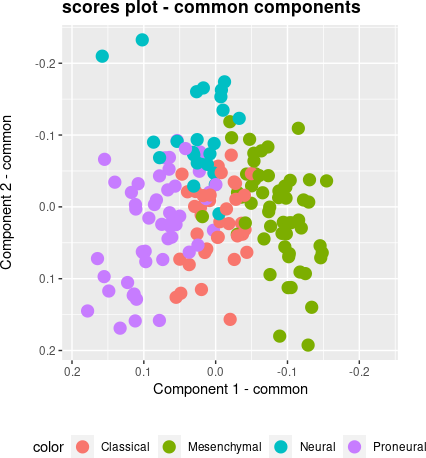# JIVE
plotRes(object=jiveRes, comps=c(1, 2), what="scores", type="common",
combined=FALSE, block=NULL, color="classname", shape=NULL, labels=NULL,
background=TRUE, palette=NULL, pointSize=4, labelSize=NULL,
axisSize=NULL, titleSize=NULL)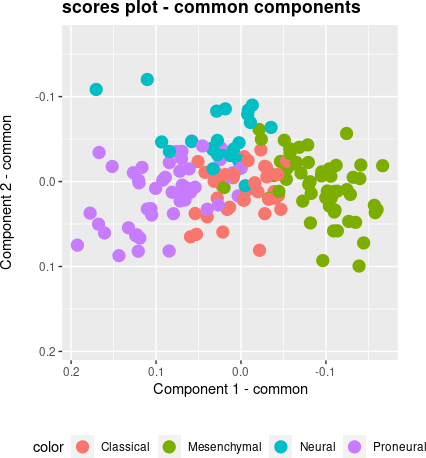In the case of the O2PLS approach, scores associated to the common components are calculated separately for each block of data. So a scatter plot of common components can be plotted independently for each block of data or together in a combined plot using the scores associated to a selected component in both blocks of data4 The function g_legend() used in these examples the commonly-used utility for sharing a legend between multiple plots. The source can be found here..

# O2PLS
# Scatterplot of scores variables associated to common components

# Associated to first block
p1 <- plotRes(object=o2plsRes, comps=c(1, 2), what="scores", type="common",
combined=FALSE, block="expr", color="classname", shape=NULL,
labels=NULL, background=TRUE, palette=NULL, pointSize=4,
labelSize=NULL, axisSize=NULL, titleSize=NULL)
# Associated to second block
p2 <- plotRes(object=o2plsRes, comps=c(1, 2), what="scores", type="common",
combined=FALSE, block="mirna", color="classname", shape=NULL,
labels=NULL, background=TRUE, palette=NULL, pointSize=4,
labelSize=NULL, axisSize=NULL, titleSize=NULL)
# Combine both plots
# g_legend function from
legend <- g_legend(p1)
grid.arrange(arrangeGrob(p1+theme(legend.position="none"),
p2+theme(legend.position="none"), nrow=1),
legend, heights=c(6/7, 1/7))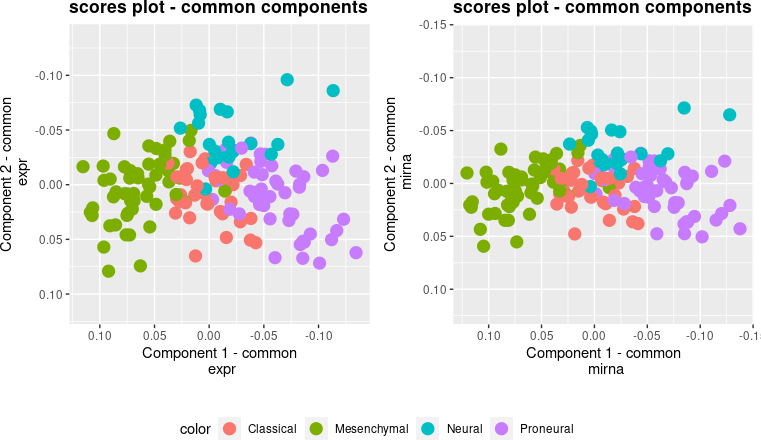# Combined plot of scores variables assocaited to common components
plotRes(object=o2plsRes, comps=c(1, 1), what="scores", type="common",
combined=TRUE, block=NULL, color="classname", shape=NULL,
labels=NULL, background=TRUE, palette=NULL, pointSize=4,
labelSize=NULL, axisSize=NULL, titleSize=NULL)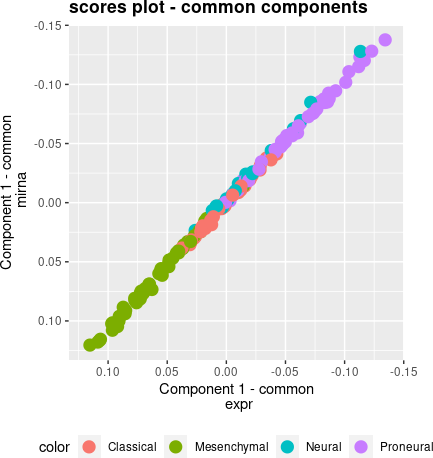The scores plot associated to individual components can be plotted using an scatterplot for each block of data in the same way that common components scores are for the O2PLS approach. A combined plot representing the components of each block of data together can be plotted, too.

# DISCO-SCA scores scatterplot associated to individual components

# Associated to first block
p1 <- plotRes(object=discoRes, comps=c(1, 2), what="scores", type="individual",
combined=FALSE, block="expr", color="classname", shape=NULL,
labels=NULL, background=TRUE, palette=NULL, pointSize=4,
labelSize=NULL, axisSize=NULL, titleSize=NULL)
# Associated to second block
p2 <- plotRes(object=discoRes, comps=c(1, 2), what="scores", type="individual",
combined=FALSE, block="mirna", color="classname", shape=NULL,
labels=NULL, background=TRUE, palette=NULL, pointSize=4,
labelSize=NULL, axisSize=NULL, titleSize=NULL)
# Combine plots
legend <- g_legend(p1)
grid.arrange(arrangeGrob(p1+theme(legend.position="none"),
p2+theme(legend.position="none"), nrow=1),
legend, heights=c(6/7, 1/7))